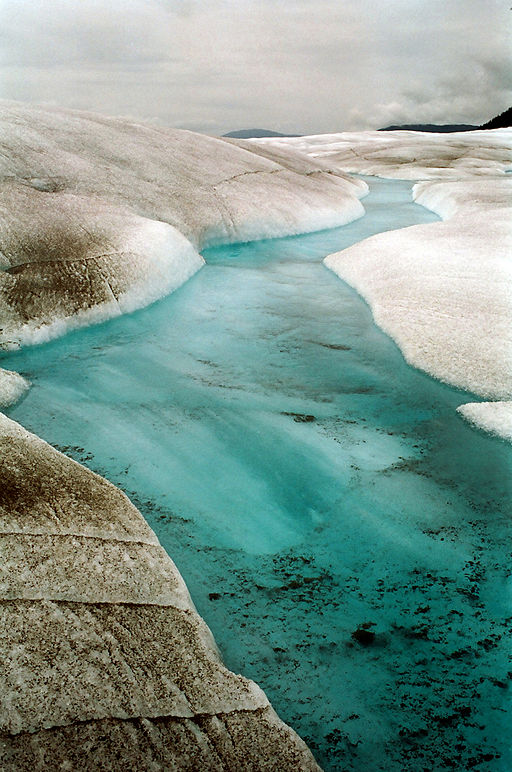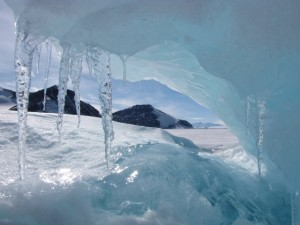# Degree day models: Modelling glacier melt

## Glacier mass balance

Numerical models of glaciers need to include an estimate of how much melting occurs on a glacier’s surface over the course of a year (e.g., Golledge and Levy1). Most of this melt (ablation) will occur during a short summer season. Understanding the relationship between glacier accumulation and glacier ablation gives a numerical modeller a value for the mass balance of a glacier. If the mass balance of a glacier is positive, the glacier will advance. If the mass balance of a glacier is negative, the glacier will shrink and recede. The mass balance at a point on a glacier can be simply estimated as:

Bn = P – R – E

Where Bn is the net balance of a glacier (expressed in metres water-equivalent), P is precipitation, R is runoff and E is evaporation2. The glacier’s average net mass balance (BN) is a function of its area (S):

BN = Bn / S

## Degree Day Model

Numerical glacier models need to be able to simplify the ablation on a glacier in order to understand a glacier’s behaviour under different climatic scenarios. One way in which the ablation on a glacier can be estimated is by using a Degree Day Model. A degree day model assumes that, for each 1°C over 0°C, a certain depth of snow (measured in millimetres water equivalent, mm w.e.) will be melted. A degree day model therefore takes into account the amount of energy available for melting over the course of the year (the total sum of daily average temperatures above 0°C over one year, the positive degree day sum or PDD), as well as how much melt occurs per degree (the degree-day factor). We will now examine each of these parameters more closely.

### Positive degree day sum (PDD)

The positive degree day sum (PDD) is the sum total of daily average temperatures above 0°C in a given time period (normally measured over one year). For example, if over the course of one week, daily temperatures are: 0°C, -2°C, 1°C, 1.5°C, -3°C, 0°C, and 2°C, then the PDD is 4.5°C for that week (1°C + 1.5°C + 2°C). Melt on snow or ice is assumed to be minimal below 0°C, so only temperatures above 0°C are added together. PDD can therefore be thought of as the total energy available for melting snow and ice over the course of one year3.

Some studies have shown that annual positive degree day sums (PDD) around the Antarctic Peninsula have increased4, resulting in changes in the onset of the melt season, melt extent and the duration of the melt season.

### Degree day factor

A degree day factor (also known as the melt coefficient1) is the amount of melt that occurs per positive degree day. Degree day factors can be measured on a glacier (for example, from ablation stakes5), and are expressed as millimetres water-equivalent per degree per day (mm w.e. °C-1 day-1). So, for a particular glacier, if the degree day factor is 2 mm w.e. °C-1 d-1, then in one day, if the average daily temperature is +1°C, then 2 mm water-equivalent of melt will be observed on ablation stakes on the glacier’s surface. If the average temperature is +2°C, then 4 mm w.e. of melt will be observed.Estimating glacier melt from air temperatures requires understanding how much snow and ice melts per degree Celsius

Melt rates per positive degree day vary geographically. Much of the variation in degree day factors is related to the differing importance of different energy balance characteristics, including wind speed and sensible heat flux5. Maritime locations, with higher wind speeds and higher humidity, have a higher degree day factor due to the latent heat of condensation and sensible heat transfer2. In dry, high-radiation, continental regions, where melt occurs through sublimation, low degree-day factors are found, because sublimation consumes high amounts of energy, meaning less energy is available for melt5. Degree day factors are also subject to significant local-scale variability, even on the same glacier. Degree day factors are also different on a specific glacier for snow and ice, which require different amounts of energy to melt. Ice is less reflective than snow, and so melts more per positive degree day.

Degree day factors are available for a wide range of glaciers, and have been compiled by several authors5,6. They range from 1-10 mm w.e. °C-1 d-1 for glaciers in the Arctic, but lower values are more typical of Antarctic regions3.

### Modelling glacier melt

A degree day model is a simple technique used to estimate or simplify the amount of melt on a glacier. Degree day models are important inputs to many numerical glacier models, including flowline models5. A degree day model (also known as a temperature index model) uses air temperature to predict the amount of melt on a glacier. A degree day model assumes an empirical relationship between melt rates and air temperatures (Positive Degree Days), and this empirical relationship (the Degree Day Factor) varies from glacier to glacier. Degree day models work well because there is a large amount of available data on air temperatures, and they perform well despite their simplicity. The calculations are simple and quick to perform, and can be easily incorporated in a more complex computer model5.

A simple degree day model assumes that, for a specific glacier6,

M = KI PDD + KS PDD

where M is the depth of snow melted (measured in millimetres water equivalent, w.e.), PDDI and PDDS are annual positive degree day sum at the specific altitude for ice and snow, and KI and KS are the degree day factors for ice and snow respectively.

Table 1. Degree day model parameters

 Parameter Symbol Units Melt (depth of snow melted) M mm w.e. Positive degree day sum per year PDD °C a-1 Degree day factor KI and KS (for ice and snow) mm w.e. d-1 °C-1

## Summary

The melt on a glacier is a function of the positive degree day sum, and the amount of melt that occurs per degree day. The depth of snow melted therefore = the degree day factor x the positive degree day sum.

The positive degree day sum is the sum of mean daily temperature for all days where the temperature is above 0°C. The degree day factor is the amount of melt (for example, as measured using an ablation stake) that occurs on a glacier per positive degree day.

Degree day models use the relationship between positive air temperatures and melt to approximate ablation for their mass balance calculations.

1.            Golledge, N.R. & Levy, R.H. Geometry and dynamics of an East Antarctic Ice Sheet outlet glacier, under past and present climates. J. Geophys. Res. 116, F03025 (2011).

2.            Benn, D.I. & Evans, D.J.A. Glaciers & Glaciation, 802 (Hodder Education, London, 2010).

3.            Huybrechts, P. & Oerlemans, J. Reponse of the Antarctic Ice Sheet to future greenhouse warming. Climate Dynamics 5, 93-102 (1990).

4.            Barrand, N.E., Vaughan, D.G., Steiner, N., Tedesco, M., Kuipers Munneke, P., van den Broeke, M.R. & Hosking, J.S. Trends in Antarctic Peninsula surface melting conditions from observations and regional climate modeling. Journal of Geophysical Research: Earth Surface 118, 315-330 (2013).

5.            Hock, R. Temperature index melt modelling in mountain areas. Journal of Hydrology 282, 104-115 (2003).

6.            Braithwaite, R.J. & Zong, Y. Sensitivity of mass balance of five Swiss glaciers to temperature changes assessed by tuning a degree-day model. Journal of Glaciology 46, 7-14 (2000).

I am a Senior Lecturer at Newcastle Univeristy, specialising in glaciology and glacial geology. I wrote and developed the AntarcticGlaciers.org website as part of an ongoing commitment to outreach, education and research impact. Read more about me at www.antarcticglaciers.org/bethan-davies.### 16 thoughts on “Degree day models: Modelling glacier melt”

1.Nina Galloway

What does “metres water-equivalent” mean and why is it not just meters?

1.Bethan Davies

Mass balance measurements on a glacier (snow gained or lost through accumulation or ablation) is measured in metres water equivalent. It represents the volume of water that would theoretically result if you melted the snowpack. This represents the average change in thickness (m gained or lost) from the glacier.

Metres water equivalent is used because snow is very variable; light, powdery snow may take up a very different volume that wet, heavy snow. Wind packing, settling under gravity, melting and refreezing all affect snow density. The measurement is calculated from the density of snow.

2.Daniella Vanella

Regarding degree days, is the “daily average” temperature calculated over a 24 hour period? Say it’s really cold at night (or morning and evening), but sunny in the day, could the average temperature be zero degrees but some melting would take place during the sunny period of the day? Would you ever use “degree hours” instead? Many thanks.

1.Bethan Davies

Positive degree days are calculated from the daily average temperature, because melting only takes place when temperatures are above 0 degrees celcius. However, some weather stations may take measurements every hour or even more frequently, and some more complicated degree day models may calculate positive degree day sums based on fractions of days (for example, see Barrand et al. 2013).

3.Marco Pedulli

Bethan, in the degree day model description just above table 1, the designations of ks and ki appear to be interchanged. A minor typo, I presume. Thanks for the informative blog, by the way.

1.Bethan Davies

Many thanks for pointing this out – I have corrected the text.
Bethan

4.Morgan Farber

In determining the loss of mm w.e. due to evaporation using this model, is there another underlying equation for the E term or is it gobbled up in the M term in the degree-day equation? Or perhaps is this determined elsewhere and added in as a constant per year?

1.Bethan Davies

The simple degree-day model accounts for evaporation within the M term. More complex energy balance models can account for these terms separately.

5.Soheb

Bethan
I am not able to find the method to estimate Degree Day factor using ablation stakes. can you suggest something?

6.Arun

Hello mam,
You have used Ki and Ks both to calculate melt depth, how anyone can know about the extent of glacier upto which Ki is applicable and on which area Ks is applicable?? if i am using temperature lapse rate and DEM to calculate temperature over glacier??
Actually i am trying to calculate Melt depth using degree day model without any field investigation except temperature data and temperature lapse rate over glacier.

1.Bethan Davies

Hello,

You could at its simplest use the ELA to differentiate between snow- and ice-covered areas perhaps, in the absence of a more complex mass-balance model?

1.Arun

But mam, temperature at ELA is low from critical temperature.
Temperature used- monthly mean 2m air temperature
ELA- Landsat images

7.Teng li

i found a sentence seems ambiguous here ‘In dry, high-radiation, continental regions, where melt occurs through sublimation, low degree-day factors are found’.
sublimation and melting are totally difference ablation process for glacier or ice sheet.how can sublimation result in melting without leaving any liquid water?

8.Parteek Singh Thind

Thank you, for this informative blog. Can you further help me by providing some literature on estimating the degree day factors using ablation stakes? I shall be very helpful to you.

9.Atif wazir

It is simple, first you should have lapse rate derived from two weather station at different altitude. second you compute temperature at every stake elevation from the lapse rate. take only those days temp which are positive for the period in which the stake is re measured. then there is simple formula already mentioned above.

10.wenhao li

Is the Greenland Degree day factors out now?

This site uses Akismet to reduce spam. Learn how your comment data is processed.